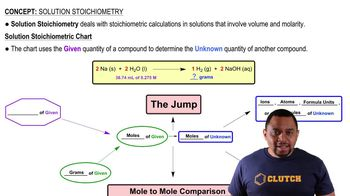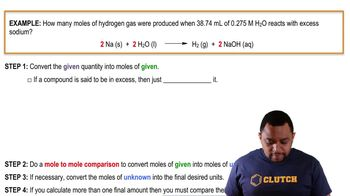Start typing, then use the up and down arrows to select an option from the list.
10:37 minutes
Problem 155
Textbook Question

# The reaction of MnO4- with oxalic acid (H2C2O4) in acidic solution, yielding Mn2+ and CO2 gas, is widely used to determine the concentration of permanganate solutions. (d) A 1.200 g sample of sodium oxalate (Na2C2O4) is dissolved in dilute H2SO4 and then titrated with a KMnO4 solution. If 32.50 mL of the KMnO4 solution is required to reach the equivalence point, what is the molarity of the KMnO4 solution? .Verified Solution
This video solution was recommended by our tutors as helpful for the problem above.
136views